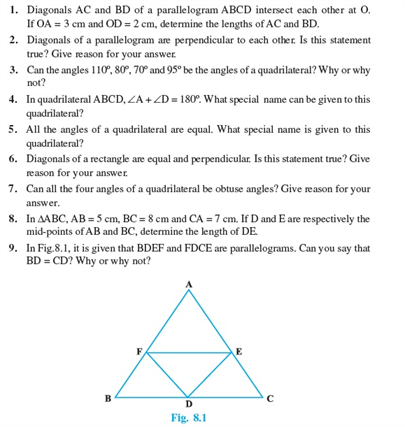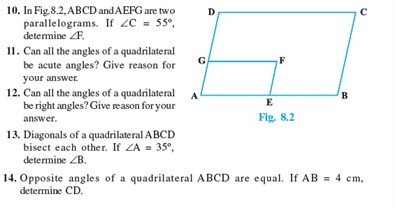# Revision Notes For CBSE Class 9 Math Chapter -8 Quadrilaterals

In geometry, a quadrilateral is defined as a polygon with four sides and four vertices. The word quadrilateral is derived from the two Latin words Quadri refers to a variant of four, and the word latus means the sides. The total sum of interior angles quadrilateral is equal to 360 degrees. Square, rhombus, rectangle, trapezium, and parallelograms are called a special type of quadrilaterals.

The formulas used for finding an area of any of the quadrilaterals is A = b * h